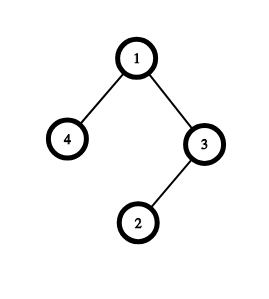Roads in a city
Tag(s):

## Data Structures, Loops, Medium, Tree

Problem
Editorial
Analytics

The layout of a city is in the form of a tree. There are $N-1$ roads connecting $N$ houses and every pair of houses has exactly one unique path between them. The distance between the two houses is equal to the number of roads in this unique path.

On the $i^{th}$ day, a member of the $(P_i) ^{th}$ house joins a company $(1 \le i, \ P_i \le N)$. A meeting of the members is held every day, and everyone, who is a member of the company until that day, is supposed to join. The meeting point is decided such that the maximum distance travelled by any of the members is as small as possible.

Your task is to help them decide the meeting point on each of the $N$ days. If multiple locations are equally convenient, then select the one that has the lowest index.

Input format

• First line: A single integer $N$ that denotes the number of houses in your city
• Next $N-1$ lines: Two space-separated integers $u, v$, that represents a bidirectional road connecting the houses $u$ and $v$
• Last line: $N$ space-separated integers that denote the array $P$. The $i ^{th}$ integer of this array denotes that the $(P_i) ^{th}$ house joined the company on the $i ^ {th}$ day.

Output format

Print the $N$ space-separated integers in a single line. The $i^{th}$ integer represents the meeting point for the meeting that has to be held on the $i^{th}$ day by satisfying all the conditions that are mentioned in the problem statement.

Constraints

$1 \le N \le 2 * 10^5$

$1 \le u, v \le N$

It is guaranteed that the road network will form a valid tree.

$1 \le P_i \le N$. All the $P_i$'s are pair-wise distinct.

For $20\%$ of the test files, $1 \le N \le 1000$

SAMPLE INPUT
4
1 3
1 4
3 2
3 2 1 4 
SAMPLE OUTPUT
3 2 3 1
ExplanationOn day $1$, only $\{3\}$ is a part of the company. The ideal meetup point is, therefore, $3$.

On day $2$, the company consists of $\{2, 3\}$. Both the locations are equally suitable for being the meetup point (Maximum distance travelled is $1$). So we choose the one with the lower index, i.e. $2$.

On day $3$, the company consists of $\{1, 2, 3\}$.  $3$ is the only ideal meetup location, since the maximum distance travelled by any of the members is $1$. (If $1$ or $2$ would have been chosen, the maximum distance would have been $2$).

On day $4$, everyone is a part of the company. $1$ and $3$ are both ideal locations (maximum distance travelled by any of the members is $2$). We choose the one with the lower index, i.e $1$.

Time Limit: 1.0 sec(s) for each input file.
Memory Limit: 256 MB
Source Limit: 1024 KB
Marking Scheme: Marks are awarded when all the testcases pass.
Allowed Languages: Bash, C, C++, C++14, Clojure, C#, D, Erlang, F#, Go, Groovy, Haskell, Java, Java 8, JavaScript(Rhino), JavaScript(Node.js), Julia, Kotlin, Lisp, Lisp (SBCL), Lua, Objective-C, OCaml, Octave, Pascal, Perl, PHP, Python, Python 3, R(RScript), Racket, Ruby, Rust, Scala, Swift, Swift-4.1, TypeScript, Visual Basic

## CODE EDITOR

Initializing Code Editor...
Your Rating:

## This Problem was Asked inChallenge Name

December Easy' 18

OTHER PROBLEMS OF THIS CHALLENGE
• Algorithms > Sorting
• Algorithms > Advanced Algorithms
• Algorithms > Dynamic Programming
• Basic Programming > Implementation
Notifications

?